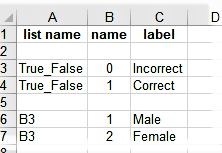# Relevance based on summation of previous answers

Hello,

I am trying to make a group relevant only if the respondent gets a certain number of previous questions right. The questions I am basing the relevance on are select_one 0/1 questions. So, for example, if I add up 6 of these questions I expect the calculate to return an integer between 0 and 6. However, I can’t seem to get the syntax right because the relevance constraint is not working as expected.

I’ve tried a few different iterations but the current calculate syntax is:

if(int((\${muw_1}) + int(\${muw_2}) + int(\${muw_3}) + int(\${muw_4}) + int(\${muw_5}) + int(\${muw_6}))>0,1,0)

I started simply with

\${muw_1} + \${muw_2}+ \${muw_3} + \${muw_4} + \${muw_5} + \${muw_6}

but then wrapped each question in the “int()” function because I read that for a 0/1 select_one question the “0” and “1” are stored as string. I also experimented with if-statements but that didn’t do any good either.

the relevance constraint I have that is based on the above calculation is:
\${muw_stop} = 1 or \${muwcorrect} = 0
Where \${muwcorrect} is the calculation and \${muw_stop} is another select_one 0/1 question. Unfortunately, I am unable to attach a sample form as a new user. Many thanks for any suggestions!

Hi @ybelyakova,

You could skip a group of questions based on the calculation values of previous questions as follows:

In the survey tab:

In the choices tab:Reference xlsform:
Group Skip.xlsx (27.4 KB)